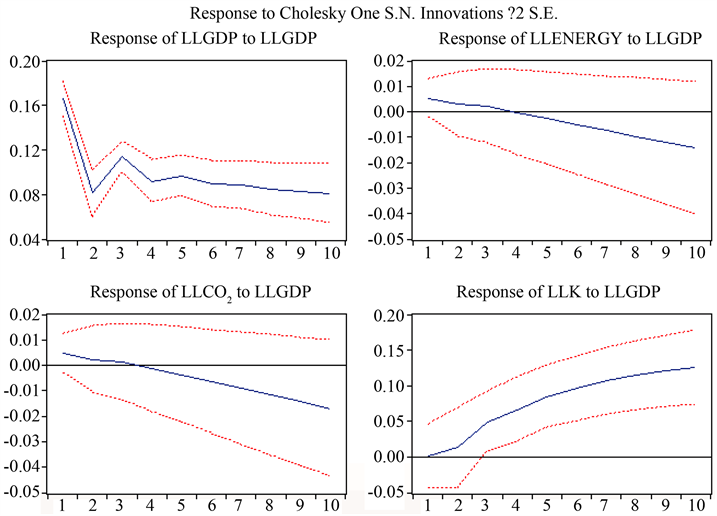# 能源消费、技术进步、经济增长对二氧化碳的动态分析Dynamic Analysis of Carbon Dioxide by Energy Consumption, Technological Progress and Economic Growth

• 全文下载: PDF(668KB)    PP.1015-1021   DOI: 10.12677/ASS.2018.77152
• 下载量: 306  浏览量: 402

This paper uses the GDP, energy consumption, technological level and CO2 data of 30 provinces and cities from 1999 to 2015 to explore the dynamic relationship between them using panel VAR model. The results show that: the increase in energy intensity promotes the increase of carbon dioxide emissions and economic growth; the increase in carbon dioxide emissions promotes economic growth and energy consumption; the improvement of technological progress inhibits the growth of carbon dioxide and energy consumption, and the relationship between them is not very obvious; the increase in economic growth has increased energy consumption, carbon dioxide, and technological progress.

1. 引言

2. 数据来源及变量的测算

2.1. 数据来源

2.2. 变量的测算

2.2.1. 二氧化碳的测算

1999年~2015年的数据来自《中国能源统计年鉴》30个省市的主要能源消耗量，中国的能源消耗被分成了9大类型，根据IPCC的《国家温室气体清单指南》(2006)中的公式计算了八大类型的每个省市的

CO2的排放量，即 ${\sum }_{j=1}^{8}{E}_{j}={\sum }_{j=1}^{8}{C}_{j}×平均低位发热量×标准煤系数×单位热值含碳量×碳氧化率$

( $j=1,2,\cdots ,8$ )，其中 ${E}_{j}$ 表示消耗第j种能源产生的二氧化碳， ${C}_{j}$ 表示第j种能源消耗量。最后一种电力的计算是根据魏思超(2012)的方法计算的  ，将其八种能源的每个省市的CO2排放量加上电力产生的CO2

2.2.2. 能源消费的测算

3. 建立模型

${Y}_{t}=\alpha +\gamma +{\beta }_{1}{Y}_{t-1}+{\beta }_{2}{Y}_{t-2}+\cdots +{\beta }_{p}{Y}_{t-p}+{\epsilon }_{t}=\alpha +\gamma +{\sum }_{i=1}^{p}{\beta }_{i}{Y}_{t-i}+{\epsilon }_{t}$ (1)

$\gamma$ 是一个 $4×1$ 的矩阵。模型中假设误差项服从N(0, ${\sigma }^{2}$ )分布，本文使用AIC，SC和HQ值达到最小的方程来确定p的值，其中  ，

$\text{AIC}=-2\frac{l}{n}+2\frac{k}{n}$ ,Table 1. Factorization of each energy source

$\text{SC}=-2\frac{l}{n}+k\frac{\mathrm{ln}n}{n}$ ,

$\text{HQ}=-2\frac{l}{n}+2k\frac{\mathrm{ln}\left(\mathrm{ln}n\right)}{n}$

n代表样本量，m表示维度， $\text{l}=-\frac{mn}{2}\left(1+\mathrm{ln}2\pi \right)-\frac{n}{2}\mathrm{ln}\left[\mathrm{det}\left({\text{Σ}}_{t},\frac{\stackrel{^}{{\epsilon }_{t}}\stackrel{^}{{\epsilon }_{t}}}{n}\right)\right]$ ，k待估参数的个数，经过实验，可以得出当滞后期p = 2时，检验AIC和SC值最小。

4. 实证分析

4.1. 单位根检验

4.2. 因果关系检验

4.3. 脉冲响应函数Figure 1. Impulse response diagram of economic growth

4.4. 方差分解

5. 结论与建议

5.1. 结论Table 4. Variance decomposition of CO2

5.2. 建议

1) 技术进步的提高可以抑制二氧化碳的排放量，因此可以引进先进的技术进行生产，但是这一方面需要权衡引进先进技术的成本。

2) 能源消耗是产生二氧化碳的主要因素，可以优化能源的使用结构以及适当的减少能源的使用量。

3) 经济增长也会促进二氧化碳的增长，所以我国应该在追求经济增长的同时，应权衡一下所产生的环境成本。

  师应来, 胡晟明. 技术进步、经济增长对二氧化碳排放的动态分析[J]. 统计与决策, 2017(16): 149-151.  姚君. 我国能源消费、二氧化碳排放与经济增长关系研究[J]. 生态经济, 2015, 31(5): 53-56.  倪超军, 马雪琴. 金融发展、技术进步与低碳经济关系的实证研究——基于面板VAR模型的动态检验[J]. 生态经济, 2017, 33(2): 41-44.  李福祥, 刘琪琦, 张霄怡. 经济增长、进出口贸易额、能源消费的动态关系研究——基于碳排放强度分组的省级面板VAR模型的实证分析[J]. 生态经济, 2016, 32(9): 75-81.  易丹辉. 数据分析与EVIEWS应用[M]. 北京: 中国人民大学出版, 2017.